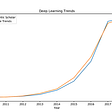# Save multiple plots to a specific path.

Intro: fairly new to matlab coming from mathematica environment.

Question: I have to calculate based on (x,y) 2D data and plot the same with the answer from the calculation shown on every plot. Assuming I have 10 2D arrays that needs to a FWHM calculation and the FWHM answer written to all of them and saved.

Code below for FWHM function:

`function width = fwhm(x,y)% Define function for FWHM at 50%  y = y / max(y); % Normalize to 1 so that half max=0.5  N = length(y); % Max length of Y  MicroscopeMag=10; % Magnification of objective  PixelWidth=7.8; % Pixel Pitch is 7.8 Microns - Hamamatsu.  %------- identifies the centre of the peak ---------------%      [~,centerindex] = max(y);% Find center peak and coordinate  %------- Identifies the last index position before half-maximum ------%  %----- Have a range containing the leading edge of the signal -----%  i = 2;  while sign(y(i)-0.5) == sign(y(i-1)-0.5) %trying to see the curve raise      i = i+1;  end  %-----Interpolate the exact point(s) where the signal's value would be 0.5-----%  %----- The next 2 lines are an attempt to determine in fractions of an  %Index exactly where the line would cross the 0.5 value.   %Simple linear interpolation -----%  interp = (0.5-y(i-1)) / (y(i)-y(i-1));   %(gives us the delta_y between the two values either side)/(shortfall)  %=linearly interpolate how far between the two index positions we should go to hit exactly 0.5  tlead = x(i-1) + interp*(x(i)-x(i-1));  % Works out the corresponding delta_x, which gives you the actual distance in terms of the timebase.  i=centerindex+1;    %------- start search for next crossing at center--------------------%  %------- Repeat process for trailing edge --------------------%  while ((sign(y(i)-0.5) == sign(y(i-1)-0.5)) && (i <= N-1))      i = i+1;  end  if i ~= N      interp = (0.5-y(i-1)) / (y(i)-y(i-1));      ttrail = x(i-1) + interp*(x(i)-x(i-1));  % Lateral Magnification x Pixel pitch of 7.8 microns.      width=((ttrail - tlead)/MicroscopeMag)*PixelWidth;   end</pre.`

Code to calculate the FWHM for every 2D array

`clear all;    a=1;    while a<=10;    filename=strcat('S',num2str(a),'.csv');    Array=csvread(filename,15,0);    x = Array(:, 1);    y = Array(:, 2);    fwhm(x,y)    a = a+1;    end`

NOTE:-

Matlabsolutions.com provide latest MatLab Homework Help,MatLab Assignment Help for students, engineers and researchers in Multiple Branches like ECE, EEE, CSE, Mechanical, Civil with 100% output.Matlab Code for B.E, B.Tech,M.E,M.Tech, Ph.D. Scholars with 100% privacy guaranteed. Get MATLAB projects with source code for your learning and research.

`for a = 1 : 10      filename = sprintf('S%d.csv', a);      Array=csvread(filename,15,0);      x = Array(:, 1);      y = Array(:, 2);      width = fwhm(x,y);      outputs = [num2cell(x), num2cell(y)];      outputs{3,1} = width;      newfilename = sprintf('Snew%d.csv', a);      xlswrite(newfilename, outputs);    end`

--

--

--

## More from Technical Source

Simple! That is me, a simple person. I am passionate about knowledge and reading. That’s why I have decided to write and share a bit of my life and thoughts to.

Love podcasts or audiobooks? Learn on the go with our new app.

## Step-in Button Changes and Alternatives## @OwlHnet: SoftwareTAP for GCLOUD and AWS.## From Good to Great: How to Become a Top-Notch Developer## Kotlin Weekly Update — 28## How do I compile MATLAB files that call Neural Network Toolbox## Technical Source

Simple! That is me, a simple person. I am passionate about knowledge and reading. That’s why I have decided to write and share a bit of my life and thoughts to.

## Deep Learning for Tabular Data## An Animated Tale of Signals and Systems## MindMaker AI Plugin for Unreal Engine 4 & 5: Blueprint Functions